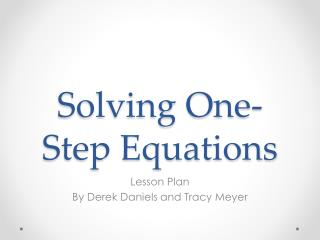DownloadDownload PresentationSolving One-Step Equations

# Solving One-Step Equations

Download Presentation## Solving One-Step Equations

- - - - - - - - - - - - - - - - - - - - - - - - - - - E N D - - - - - - - - - - - - - - - - - - - - - - - - - - -
##### Presentation Transcript

1. Solving One-Step Equations Lesson Plan By Derek Daniels and Tracy Meyer

2. Subject • Primary Subject – Math • Secondary Subject – Algebra • Grade Level – 9th grade • Using basic algebra equations in everyday situations

3. Lesson Objectives & Goals • To learn the first steps in solving polynomial equations using 1 unknown variable. • Goal: all students will participate in activities and have practice completing problems before the end of the lesson.

4. Sunshine State Standard MA.912.A.3.1 Solve linear equations in one variable that include simplifying algebraic expressions

5. List of Resources • PowerPoint • Smartboard • Internet • YouTube • Games • Calculator • Document Reader • Hand Held Calculator

6. References • Ijustwannajam (poster). (October 3, 2009). Why math is important [video]. Retrieved from http://www.youtube.com/watch?v=gDkd0Vaxf-c&feature=player_detailpage • Popovici, Doina (producer). (October 22, 2008). Hoop Shoot [game]. Retrieved from http://www.math-play.com/One-Step-Equation-Game.html • Larson, R., Boswell, L., Kinold, T.D., & Stiff, L. (2011). Algebra 2, 162-169.

7. Solving One-Step Equations Algebra One Chapter 3 Lesson 1

8. Variables • In algebraic expressions, letters represent variables. These letters are actually numbers in disguise. They can be any letter and represent an unknown.

9. Why Math is Important http://www.youtube.com/watch?v=gDkd0Vaxf-c&feature=player_detailpage

10. X-5=3 X+5=8

12. Addition Property of Equality X-a=b x-a+a=b+a x=b+a X-5=3 x-5+5=3+5 x=3+5

13. Subtraction Property of Equality

14. Subtraction Property of Equality X+a=bx+a-a=b-ax=b-a X+5=8x+5-5=8-5x=8-5

15. Lesson Notes

16. Practice Problems Solve each equation. Check your solution.

17. Game http://www.math-play.com/One-Step-Equation-Game.html

18. HOMEWORK Page 137 1-37 odd 54-61 all